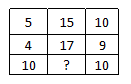# Missing Number Problems for SSC CGL PDF

0
16460

Missing Number Problems for SSC CGL PDF:

Question 1:In the following question, select the missing number from the given seriesa) 11
b) 12
c) 15
d) 10

Question 2: In the following question, select the missing number from the given series.

a) 9
b) 8
c) 16
d) 12

Question 3: In the following question select the missing number from the given series

a) 37
b) 47
c) 48
d) 25

Question 4: In the following question, select the missing number from the given series

a) 9
b) 4
c) 8
d) 2

Question 5: In the following question select the missing number from the given series

a) 44
b) 48
c) 50
d) 64

Question 6: In the following question, select the missing number from the given series ?

a) 0
b) 4
c) 2
d) 6

Question 7: Find the missing number as per given Series in the table

a) 35
b) 45
c) 25
d) 50

Question 8: In the following question select the missing number of the given series

a) 9
b) 8
c) 6
d) 7
Question 9: In the following questions select the missing number from the given series.

a) 81
b) 361
c) 289
d) 225

Question 10: In the following question, select the missing number from the given series

a) 119
b) 120
c) 170
d) 190

The sum of three digits in the horizontal row is equal to 30
1) 5 + 15 + 10 = 30
2) 4 + 17 + 9 = 30
3) 10 + x + 10 = 30 ; x = 10
Hence, option D is the correct answer.

The pattern followed is that the number in the last row is obtained by multiplying the other two.
Eg :- $3\times7=21$
$5\times6=30$
Similarly, $8\times1=8$
=> Ans – (B)

The logic followed here is,

$\sqrt{49}$ + $\sqrt{81}$ $\Rightarrow$ 7 + 9 = 16,

$\sqrt{169}$ + $\sqrt{144}$ $\Rightarrow$ 13 + 12 = 25,

$\sqrt{484}$ + $\sqrt{624}$ $\Rightarrow$ 22 + 25 = 47.

Hence, option B is the correct answer.

The pattern followed for $a,b,c$ and $d$ in each column is : $a=(c\times d)-b$

Eg :- $(3\times5)-6=15-6=9$

$(3\times4)-7=12-7=5$

Similarly, $(x\times7)-6=8$

=> $7x=8+6=14$

=> $x=\frac{14}{7}=2$

=> Ans – (D)

In each column, the number at the end is the average of other two.

Eg :- $\frac{(36+72)}{2}=\frac{108}{2}=54$

$\frac{(44+88)}{2}=\frac{132}{2}=66$

Similarly, $\frac{(32+64)}{2}=\frac{96}{2}=48$

=> Ans – (B)

The pattern followed is that in each column, the number at the middle is obtained by multiplying the other two.

Eg :- $12\times9=108$

$9\times8=72$

Similarly, $9\times x=36$

=> $x=\frac{36}{9}=4$

=> Ans – (B)

The pattern followed is that in each row, the number at the middle is obtained by multiplying the other two.

Eg :- $6\times7=42$

$8\times4=32$

Similarly, $9\times5=45$

=> Ans – (B)

The sum of each column is same.

Eg :- $6+7+3=16$

and $10+2+4=16$

Similarly, $9+1+x=16$

=> $x=16-10=6$

=> Ans – (C)

In each row, the number in the middle is the square of the first number.

Eg :- $13^2=169$ and $12^2=144$

Similarly, $15^2=225$

=> Ans – (D)

The pattern followed is that in each row the number at the end is the square root of the product of first two.

Eg :- $\sqrt{25\times144}=\sqrt{5\times5\times12\times12}=5\times12=60$

$\sqrt{81\times225}=\sqrt{9\times9\times15\times15}=9\times15=225$

Similarly, $\sqrt{49\times289}=\sqrt{7\times7\times17\times17}=7\times17=119$

=> Ans – (A)